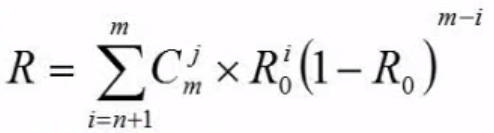• 并联系统 仅当所有子系统都失效的时候该并联系统才会失效，所以改并联系统的失效率或者可靠性很好计算，就是用1 - 失效率 即可。比如说有一个系统是由三个并联的环节组成，那么当且仅当三个环节全部失效的时候，才会...
串联系统 每个环节都必须能够正常工作 所以串联系统的可靠度就是 R0的正常率 * R1的正常率 * R2的正常率 * R3的正常率 一直到结束
并联系统 仅当所有子系统都失效的时候该并联系统才会失效，所以改并联系统的失效率或者可靠性很好计算，就是用1 - 失效率 即可。比如说有一个系统是由三个并联的环节组成，那么当且仅当三个环节全部失效的时候，才会失效，因此可靠性就是1 - 三个环节全部失效的概率 也就是 1 - (1-R0成功的概率)（1-R1成功的概率）（1-R2成功的概率）
模冗余系统和混合系统。 冗余系统的目的 其实是为了提高系统的可靠性 那么是如何冗余的呢 也很好理解 就是让原本一个环节的系统再并联上几个环节，且每个环节的职能和工作原理是一样的，每个环节再将计算后的结果交给一个表决器，由表决器来抉择到底选则哪个结果。公式如下将串并联系统同时使用的时候 ，就先把并联的可靠性计算了，再把串联的可靠性计算了，就行
展开全文• 设一个系统由三个相同子系统并联构成，子系统的可靠性为0. 9，平均无故障时间为10000小时，则系统可靠性为(2)和平均无故障时间为(3)。(2) A.0.729 B. 0.9 C. 0.999 D. 0.99(3) A.1. 9999 B. 18000 C. 9000 D. 18333...

设一个系统由三个相同子系统并联构成，子系统的可靠性为0. 9，平均无故障时间为10000小时，则系统可靠性为(2)和平均无故障时间为(3)。
(2) A.0.729 B. 0.9 C. 0.999 D. 0.99
(3) A.1. 9999 B. 18000 C. 9000 D. 18333
参考答案：C、D
计算机系统是一个复杂的系统，而且影响其可靠性的因素也非常繁复，很难直接对其进行可靠性分析;但通过建立适当的数学模型，把大系统分割成若干子系统，可以简化其分析过程。常见的系统可靠性数学模型有以下二种:
1)串联系统:假设一个系统由n个子系统组成，当且仅当所有的子系统都有能正常工作时，系统才能正常工作，这种系统称为串联系统
设系统各个子系统的可靠性分别用R1, R2, R3……, Rn表示，则系统的可靠性
R=R1×R2×R3×……×Rn
如果系统的各个子系统的失效率分别用λ1, λ2, λ3……, λn来表示，则系统的失效率
λ=λ1+λ2+λ3+……+λn
假设本题三个子系统是串联的，n=3，R1=R2=R3=0.9，λ1=λ2=λ3=1/10000=0.0001，则：
系统可靠性　　　　　　R= R1×R2×R3=0.9×0.9×0.9=0.729
系统失效率　　　　　　λ=λ1+λ2+λ3=0.0001+0.0001+0.0001=0.0003
系统平均故障间隔时间　MTBF= = = =3333
(2)并联系统:假如一个系统由n个子系统组成，只要有一个子系统能够正常工作，系统就能正常工作，
设系统各个子系统的可靠性分别用R1, R2, R3……, Rn表示，则系统的可靠性
R=1-(1-R1)×(1-R2)×(1-R3)×……×(1-Rn)
如果系统的各个子系统的失效率分别用λ1, λ2, λ3……, λn来表示，则系统的失效率
λ= 1/((1/1*λ1)+(1/2*λ2)+(1/3*λ3)+...+(1/n*λn)) 网上资料不是太全，我现在补上
根据本题题意可知，n=3，R1=R2=R3=0.9，λ1=λ2=λ3=1/10000=0.0001，则：
系统可靠性　　　　　　R= (1-R1)×(1-R2)×(1-R3)=1-(1-0.9)×(1-0.9)×(1-0.9)=0.999
系统失效率　　　　　　λ=1/(1/0.0001+1/0.0002+1/0.0003)= 5.4545E-5网上这个答案也是错的
系统平均故障间隔时间　MTBF= = = =18333
所以本题正确答案为：(2) C (3)D

展开全文• 题目：设一个系统由三个相同子系统并联构成，子系统的可靠性为0. 9，平均无故障时间为10000小时，则系统可靠性为(2)和平均无故障时间为(3)。(2) A.0.729 B. 0.9 C. 0.999 D. 0.99(3) A.1. 9999 B. 18000 C. 9000 D. ...

题目：设一个系统由三个相同子系统并联构成，子系统的可靠性为0. 9，平均无故障时间为10000小时，则系统可靠性为(2)和平均无故障时间为(3)。
(2) A.0.729 B. 0.9 C. 0.999 D. 0.99
(3) A.1. 9999 B. 18000 C. 9000 D. 18333
计算机系统是一个复杂的系统，而且影响其可靠性的因素也非常繁复，很难直接对其进行可靠性分析;但通过建立适当的数学模型，把大系统分割成若干子系统，可以简化其分析过程。常见的系统可靠性数学模型有以下三种:
1)串联系统:假设一个系统由n个子系统组成，当且仅当所有的子系统都有能正常工作时，系统才能正常工作，这种系统称为串联系统，如图1所示。设系统各个子系统的可靠性分别用R1, R2, R3……, Rn表示，则系统的可靠性
R=R1×R2×R3×……×Rn
如果系统的各个子系统的失效率分别用λ1, λ2, λ3……, λn来表示，则系统的失效率
λ=λ1+λ2+λ3+……+λn
则系统平均故障间隔时间为：
MTBF=1/λ
假设本题三个子系统是串联的，n=3，R1=R2=R3=0.9，λ1=λ2=λ3=1/10000=0.0001，则：
系统可靠性　R= R1×R2×R3=0.9×0.9×0.9=0.729
系统失效率　λ=λ1+λ2+λ3=0.0001+0.0001+0.0001=0.0003
系统平均故障间隔时间　MTBF=1/0.0003=3333
(2)并联系统:假如一个系统由n个子系统组成，只要有一个子系统能够正常工作，系统就能正常工作，如图2所示。设系统各个子系统的可靠性分别用R1, R2, R3……, Rn表示，则系统的可靠性
R=1-(1-R1)×(1-R2)×(1-R3)×……×(1-Rn)
如果系统的各个子系统的失效率均为λ，则系统的失效率μ为则系统平均故障间隔时间为：
MTBF= 1/μ
根据本题题意可知，n=3，R1=R2=R3=0.9，λ1=λ2=λ3=1/10000=0.0001，则：
系统可靠性　　　　　　R = (1-R1)×(1-R2)×(1-R3)=1-(1-0.9)×(1-0.9)×(1-0.9)=0.999
系统失效率　　　　　　μ = 1/((1/0.0001)*(1/1+1/2+1/3))=6/(10000*11)
系统平均故障间隔时间　MTBF=10000*11/6=18333
所以本题正确答案为：(2) C (3)D
注：以上资料(包括图片)来源于互联网，版权归原作者所有。

展开全文• 设一个系统由三个相同子系统并联构成，子系统的可靠性为0.9，平均无故障时间为10000小时，则系统可靠性为(2)和平均无故障时间为(3)。(2) A.0.729 B. 0.9 C. 0.999 D. 0.99(3) A.1. 9999 B. 18000 C. 9000 D. 18333...

设一个系统由三个相同子系统并联构成，子系统的可靠性为0.
9，平均无故障时间为10000小时，则系统可靠性为(2)和平均无故障时间为(3)。
(2) A.0.729 B. 0.9 C. 0.999 D. 0.99
(3) A.1. 9999 B. 18000 C. 9000 D. 18333
试题分析　　C　D
计算机系统是一个复杂的系统，而且影响其可靠性的因素也非常繁复，很难直接对其进行可靠性分析;但通过建立适当的数学模型，把大系统分割成若干子系统，可以简化其分析过程。常见的系统可靠性数学模型有以下三种:
1)串联系统:假设一个系统由n个子系统组成，当且仅当所有的子系统都有能正常工作时，系统才能正常工作，这种系统称为串联系统，如图1所示。
设系统各个子系统的可靠性分别用R1, R2, R3……, Rn表示，则系统的可靠性
R=R1×R2×R3×……×Rn
如果系统的各个子系统的失效率分别用λ1, λ2, λ3……, λn来表示，则系统的失效率
λ=λ1+λ2+λ3+……+λn
则系统平均故障间隔时间为：
MTBF= =
假设本题三个子系统是串联的，n=3，R1=R2=R3=0.9，λ1=λ2=λ3=1/10000=0.0001，则：
系统可靠性　　　　　　R= R1×R2×R3=0.9×0.9×0.9=0.729
系统失效率　　　　　　λ=λ1+λ2+λ3=0.0001+0.0001+0.0001=0.0003
系统平均故障间隔时间　MTBF= = = =3333
(2)并联系统:假如一个系统由n个子系统组成，只要有一个子系统能够正常工作，系统就能正常工作，如图2所示。
设系统各个子系统的可靠性分别用R1, R2, R3……, Rn表示，则系统的可靠性
R=1-(1-R1)×(1-R2)×(1-R3)×……×(1-Rn)
如果系统的各个子系统的失效率分别用λ1, λ2, λ3……, λn来表示，则系统的失效率
λ=
则系统平均故障间隔时间为：
MTBF=
当N=2时，MTBF＝
设λ1=λ2=λ3=……=λn =λ，则MTBF==
根据本题题意可知，n=3，R1=R2=R3=0.9，λ1=λ2=λ3=1/10000=0.0001，则：
系统可靠性　　　　　　R=
(1-R1)×(1-R2)×(1-R3)=1-(1-0.9)×(1-0.9)×(1-0.9)=0.999
系统失效率　　　　　　λ= =5.4545
系统平均故障间隔时间　MTBF= = = =18333
所以本题正确答案为：(2) C (3)D

展开全文• 计算机组成原理-计算机可靠性模型 计算机系统是一个复杂的系统，影响可靠性的因素非常多，难以进行直接可靠性分析，但通过建立...可靠性R计算 假设各个子系统的可靠性分别用R1R2…表示，则系统可靠性为 R=R1R2...R...计算机组成原理
• 题目：设一个系统由三个相同子系统并联构成，子系统的可靠性为0. 9，平均无故障时间为10000小时，则系统可靠性为(2)和平均无故障时间为(3)。 (2) A.0.729 B. 0.9 C. 0.999 D. 0.99 (3) A.1. 9999 B. 18000 C. 9000 D...
• ## 系统可靠性计算

万次阅读 2019-04-27 17:32:05
可靠性计算主要涉及三种系统，即串联系统、并联系统和冗余系统，其中串联系统和并联系统的可靠性计算都非常简单，只要了解其概念，公式很容易记住。冗余系统要复杂一些。在实际的考试当中，考得最多的就是串并混合...
• 可靠性计算公式大全》由会员分享，可在线阅读，更多相关《可靠性计算公式大全(3页珍藏版)》请在人人文库网上搜索。1、计算机系统的可靠性是制从它开始运行(t=0)到某时刻t这段时间内能正常运行的概率，用R(t)表示 ...
• 一、可靠性计算（串联系统、并联系统以及他们的组合） 各个子系统的可靠性分别用R1、R2……Rn，失效率（一个系统的失效率与该系统平均故障时间（MTBF）在数值上是成倒数的）分别用λ1、λ2、……λn表示 串联系统...计算机系统 可靠性
• ## 计算机可靠性计算

千次阅读 2012-07-25 15:57:14
计臬机可靠性计算分为:串联系统和关联系统两种   串联系统计算公式:R=R1*R2*R3......RN   例如:设计算机系统由CPU,存偖器,I/O三部分组成,其可靠性分别为0.95,0.9,0.85.求计算机的可靠性 解: R = R1 * R2 * R3...
• 串联模型可靠计算 R = R1 X R2 X R3 X … X Rn 比如R1,R2,R3都是0.9，则R=0.93 = 72.9% 串联模型失效率计算 失效率有可能是会大于1的，它只是一个简化公式，当失效率极低的时候可以快速计算结果。 并联系统 并联...程序人生
• 设一个系统由三个相同子系统并联构成，子系统的可靠性为0. 9，平均无故障时间为10000小时，则系统可靠性为(2)和平均无故障时间为(3)。(2) A.0.729 B. 0.9 C. 0.999 D. 0.99(3) A.1. 9999 B. 18000 C. 9000 D. 18333...c
• https://www.bilibili.com/video/av19665344/?p=19
• 为了有效处理载荷共享并联系统的可靠性问题，首先分析了并联系统的相关失效，并分步计算并联系统可靠度，应用全概率公式和Miner理论对并联系统进行了疲劳累积损伤可靠性建模。其次，运用Monte Carlo方法对零件寿命...
• 论文研究-并联系统的模糊可靠性.pdf, 本文在模糊可靠性理论的基础上,应用模糊数学的原理和方法,建立了一套计算并联系统模糊可靠性的公式,并对一个实例进行了计算
• 　可靠性计算主要涉及三种系统，即串联系统、并联系统和冗余系统，其中串联系统和并联系统的可靠性计算都非常简单，只要了解其概念，公式很容易记住。冗余系统要复杂一些。在实际的考试当中，考得最多的就是串并混合...
• 在开关不完全可靠且开关有优先修理权的情况下,研究n个同型部件并联系统。建立了部件及开关的工作寿命和维修时间均服从指数分布的数学模型,给出了系统可靠性指标的计算方法及具体结果。
• 为了使并联系统失效概率估计值的方差大大减小，依据并联系统重要方向的定义和单模式线抽样方法的基本原理，建立了适用于并联系统可靠性分析的马尔可夫链线抽样方法，给出了并联系统马尔可夫链线抽样方法失效概率估算...
• 电子行业的小伙伴们都知道UPS，UPS即叫不间断电源，它是将蓄电池与主机相连接，并且通过主机逆变器等模块电路将直流电转换成市电的系统设备。UPS主要是用于给单台计算机、计算机网络系统或其它电力电子设备如电磁阀...
• 可靠性计算主要涉及三种系统，即串联系统、并联系统和冗余系统，其中串联系统和并联系统的可靠性计算都非常简单，只要了解其概念，公式很容易记住。冗余系统要复杂一些。在实际的考试当中，考得最多的就是串并混合...
• 计算机系统的可靠性从它开始运行(t=0)到某时刻t这段时间内能正常运行的概率，用R(t)表示。失效率指单位时间内失效的元件数与元件总数的比例，以λ表示，当λ为常数时，可靠性与失效率的关系为: R(t)=е^(-λt)，指数...
• 工业统计第六章可靠性分析现代工业统计 第6章 可靠性分析2 第6章 可靠性分析 概 述 §6.1 从可靠性理论产生发展应用谈起 §6.2 可靠度、失效率与寿命分布 §6.3 系统的可靠性 §6.4 寿命试验与可靠度的非参数估计 §...
• 1）串联系统假设一个系统由n个子系统组成，当所有的子系统都有能正常工作时，系统才能正常工作，这种系统称为串联系统，如下图设系统各个子系统的可靠性分别用R1，R2，...，Rn表示，则系统的可靠性为： R=R1×R2×.....
• 　可靠性计算主要涉及三种系统，即串联系统、并联系统和冗余系统，其中串联系统和并联系统的可靠性计算都非常简单，只要了解其概念，公式很容易记住。冗余系统要复杂一些。在实际的考试当中，考得最多的就是串并混合...服务器 c
• 串联系统的可靠性R=R1R2…RN; (R1,R2,RN 是各个子系统的可靠性。) 并联系统的可靠性R=1-（1-R1）（1-R2）…(1-RN)...